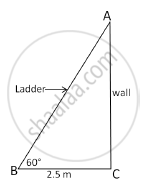# A ladder leaning against a wall makes an angle of 60° with the horizontal. If the foot of the ladder is 2.5 m away from the wall, find the length of the ladder - Mathematics

A ladder leaning against a wall makes an angle of 60° with the horizontal. If the foot of the ladder is 2.5 m away from the wall, find the length of the ladder

#### Solution

Let AB be the ladder and CA be the wall.

The ladder makes an angle of 60° with the horizontal.

∴ΔABC is a 30°-60°-90°, right triangle.

Given: BC = 2.5 m, ∠ABC = 60°

∴AB = 5 cm and ∠BAC = 30°

From Pythagoras Theorem, we have

AB2 = BC2 + CA2

∴52 = (2.5)2 + (CA)2

∴(CA)2 = 25 – 6.25 = 18.75 m

Hence, length of the ladder is  sqrt18.75~~4.33mConcept: Right-angled Triangles and Pythagoras Property
Is there an error in this question or solution?